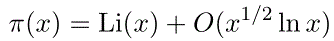# Riemann hypothesis

Riemann noted that his zeta function had trivial zeros at -2, -4, -6, ... and that all nontrivial zeros were symmetric about the line Re(s) = 1/2. The Riemann hypothesis is that all nontrivial zeros are on this line. In fact the classical proofs of the prime number theorem require an understanding of the zero free regions of this function, and in 1901 von Koch showed that the Riemann hypothesis is equivalent to:Because of this relationship to the prime number theorem, Riemann's hypothesis is easily one of the most important conjectures in prime number theory.